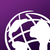# Problem with unsuspected coordinate values when creating polygons with arcpy.

285
3
01-24-2020 04:37 AMNew Contributor

I have a problem when creating polygon features with arcpy. I want to save rounded X and Y vertices for example to two decimal places. Example:

I have coordinates given on two decimal places. I am expecting rounding error due to limitations in precision of float data type.

Lets say we are working with projected coordinate system with base units of 1 Meter.

Example script (using dummy coordinate values):

import arcpy

point_coord = arcpy.Point(1.10, 2.13)

point = arcpy.PointGeometry(point_coord)

linestring_coords = [arcpy.Point(1.10, 2.13), arcpy.Point(2.44, 5.12)]

ls_array = arcpy.Array(linestring_coords)

linestring = arcpy.Polyline(ls_array)

poly_coords = [arcpy.Point(1.10, 2.13), arcpy.Point(2.44, 5.12), arcpy.Point(0.32, 4.2), arcpy.Point(1.10, 2.13)]

p_array = arcpy.Array(arcpy.Array(poly_coords))

poly = arcpy.Polygon(p_array)

print("Constructed point: {}".format(point.WKT))

print("Constructed polyline: {}".format(linestring.WKT))

print("Constructed polygon: {}".format(poly.WKT))

Result:

Constructed point: POINT (1.1000000000000001 2.1299999999999999)

Constructed polyline: MULTILINESTRING ((1.1000000000000001 2.1299999999999999, 2.4399999999999999 5.1200000000000001))

Constructed polygon: MULTIPOLYGON (((1.10009765625 2.130126953125, 2.44012451171875 5.1201171875, 0.32012939453125 4.2000732421875, 1.10009765625 2.130126953125)))

As said I am expecting rounding errors on for the values in point and polyline. But error on polygon coordinates is a lot bigger than 10e-10. If we say that base projection units are in meters that brings an error of 0.1 mm which is a lot in some surveying applications. I know that this error can be virtually rounded to 2 decimal places in arcmap or some other, but if I want to take these coordinates programically and perform some calculations the error grows and stays in data.

Is there a way to enforce rounding to values of coordinates for polygon features. How can this be the case in software meant for managing acurrate and high quality data.

I know for a fact, that some libraries outside arcgis ecosystem round coordinates normally for polygons (like point and polyline above).

How can I achieve same 'precision' as when creating polylines but with Polygons.

Tags (3)
3 RepliesbyMVP Esteemed Contributor

There are issues if you don't use a defined coordinate system for your data.  I didn't see one specified.

specify it when creating the Polygon object

poly = arcpy.Polygon(arcpy.Array(aa), SR)

where aa is a list or array of points and SR is a spatial reference object (name, of code is also fine)New Contributor

Thank you for your fast anwser!!

I can see what you mean. I didn't think of defining coordinate system for each geometry object since feature class I'm inserting in (in other solution) has spatial reference defined. This fixed the accuracy a lot but it is still not the same as with polyline if we compare first two vertices.

Example:

import arcpy

sr = arcpy.SpatialReference(3857) #projected coordinate system

point_coord = arcpy.Point(1.10, 2.13)

point = arcpy.PointGeometry(point_coord, sr)

linestring_coords = [arcpy.Point(1.10, 2.13), arcpy.Point(2.44, 5.12)]

ls_array = arcpy.Array(linestring_coords)

linestring = arcpy.Polyline(ls_array, sr)

poly_coords = [arcpy.Point(1.10, 2.13), arcpy.Point(2.44, 5.12), arcpy.Point(0.32, 4.2), arcpy.Point(1.10, 2.13)]

p_array = arcpy.Array(arcpy.Array(poly_coords))

poly = arcpy.Polygon(p_array, sr)

print("Constructed point: {}".format(point.WKT))

print("Constructed polyline: {}".format(linestring.WKT))

print("Constructed polygon: {}".format(poly.WKT))

Results:

Constructed point: POINT (1.1000000000000001 2.1299999999999999)

Constructed polyline: MULTILINESTRING ((1.1000000000000001 2.1299999999999999, 2.4399999999999999 5.1200000000000001))

Constructed polygon: MULTIPOLYGON (((1.1000000014901161 2.1299999989569187, 2.4400000013411045 5.1200000010430813, 0.32000000029802322 4.1999999992549419, 1.1000000014901161 2.1299999989569187)))byExplore the ArcPy classes for more goodies